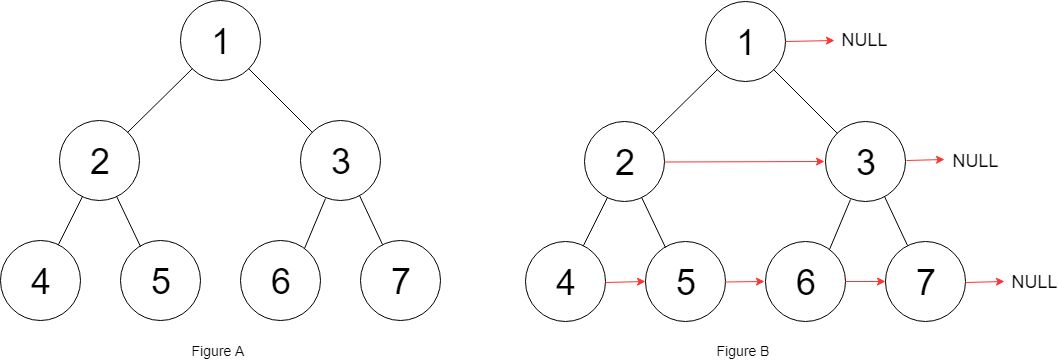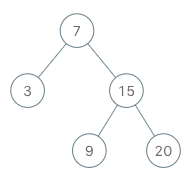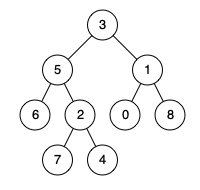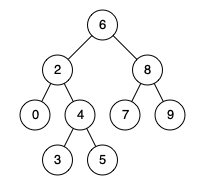# Backtracking - 47. Permutations II

47. Permutations II Given a collection of numbers that might contain duplicates, return all possible unique permutations. Example: Input: [1,1,2] Output: [ [1,1,2], [1,2,1], [2,1,1] ] 思路： 和46题差别在于数字有重复，需要返回unique的组合，组合问题，只要有重复，通解思路都是先先排序，剩下的就基本和46题一样，还需判断一个数是否被用过来剪枝。 代码： go： func permuteUnique(nums []int) [][]int { sort.Ints(nums) return permute(nums) } func permute(nums []int) [][]int { var res [][]int dfs(0, nums, &res) return res } func dfs(start int, nums []int, res *[][]int){ if....# Backtracking - 46. Permutations

46. Permutations Given a collection of distinct integers, return all possible permutations. Example: Input: [1,2,3] Output: [ [1,2,3], [1,3,2], [2,1,3], [2,3,1], [3,1,2], [3,2,1] ] 思路： 题目意思很简单就是求出一个数组的排列组合，思路就是使用回溯来做。 代码： go： func permute(nums []int) [][]int { var res [][]int dfs(0, nums, &res) return res } func dfs(start int, nums []int, res *[][]int){ if start == len(nums) { *res = append(*res, append([]int{}, nums...)) return } for i := start; i < len(nums); i++ { num....# Dynamic Programming - 377. Combination Sum IV

377. Combination Sum IV Given an integer array with all positive numbers and no duplicates, find the number of possible combinations that add up to a positive integer target. Example: nums_ = [1, 2, 3] target = 4 The possible combination ways are: (1, 1, 1, 1) (1, 1, 2) (1, 2, 1) (1, 3) (2, 1, 1) (2, 2) (3, 1) Note that different sequences are counted as different combinations. Therefore the output is 7. Follow up: What if negative numbers are allowed in the given array? How does it change....# Backtracking - 216. Combination Sum III

216. Combination Sum III Find all possible combinations of k numbers that add up to a number n, given that only numbers from 1 to 9 can be used and each combination should be a unique set of numbers. Note: All numbers will be positive integers. The solution set must not contain duplicate combinations. Example 1: Input: k = 3, n = 7 Output: [[1,2,4]] Example 2: Input: k = 3, n = 9 Output: [[1,2,6], [1,3,5], [2,3,4]] 思路： 这一题是组合系列的变形题，要求用k个1-9的数组组合出n值，采用回溯来做，使用组合的个数为k个和为n来剪....# Backtracking - 40. Combination Sum II

40. Combination Sum II Given a collection of candidate numbers (candidates) and a target number (target), find all unique combinations in candidates where the candidate numbers sums to target. Each number in candidates may only be used once in the combination. Note: All numbers (including target) will be positive integers. The solution set must not contain duplicate combinations. Example 1: Input: candidates = [10,1,2,7,6,1,5], target = 8,....# Backtracking - 39. Combination Sum

39. Combination Sum Given a set of candidate numbers (candidates) (without duplicates) and a target number (target), find all unique combinations in candidates where the candidate numbers sums to target. The same repeated number may be chosen from candidates unlimited number of times. Note: All numbers (including target) will be positive integers. The solution set must not contain duplicate combinations. Example 1: Input: candida....# Backtracking - 77. Combinations

77. Combinations Given two integers n and k, return all possible combinations of k numbers out of 1 … n. Example: Input: n = 4, k = 2 Output: [ [2,4], [3,4], [2,3], [1,2], [1,3], [1,4], ] 思路： 回溯思想中的组合问题系列，题目意思很明确，问你1~n内挑k个数组合起来，有多少种方式。使用dfs求解，一直找到临时结果中有k个数，之后回溯。 代码： go： func combine(n int, k int) [][]int { var res [][]int dfs(n, 1, k, []int{}, &res) return res } func dfs(n, start, k int, temp []int, res*[][]int) { if k == 0 { *res = append(*res, app....# Backtracking - 90. Subsets II

90. Subsets II Given a collection of integers that might contain duplicates, nums, return all possible subsets (the power set). Note: The solution set must not contain duplicate subsets. Example: Input: [1,2,2] Output: [ , , [1,2,2], [2,2], [1,2], [] ] 思路： 于78题相比，这题的数组中的元素可能重复，其实可以先对数组排序，然后再用dfs求解。 代码： go： func subsetsWithDup(nums []int) [][]int { res := [][]int{[]int{}} sort.Ints(nums) doSubsetsWithDup(nums, []int{}, &res) return res } func doSubsetsWithDup(nums []in....# Backtracking - 78. Subsets

78. Subsets Given a set of distinct integers, nums, return all possible subsets (the power set). Note: The solution set must not contain duplicate subsets. Example: Input: nums = [1,2,3] Output: [ ,   ,   ,   [1,2,3],   [1,3],   [2,3],   [1,2],   [] ] 思想： 求出一个组数的子集，这算是回溯当中的最基本的入门题目，感觉地位相当于dp当中的斐波那契。这里使用dfs求解，然后不断往回回溯求解。 代码： go： func subsets(nums []int) [][]int { var res [][]int backtrack(nums, 0, []int{}, &res) return re....# Tree - 331. Verify Preorder Serialization of a Binary Tree

331. Verify Preorder Serialization of a Binary Tree One way to serialize a binary tree is to use pre-order traversal. When we encounter a non-null node, we record the node’s value. If it is a null node, we record using a sentinel value such as #. _9_ / \ 3 2 / \ / \ 4 1 # 6 / \ / \ / \ # # # # # # For example, the above binary tree can be serialized to the string "9,3,4,#,#,1,#,#,2,#,6,#,#", where # represents a null node. Given a string of comma separated values, veri....# Tree - 95. Unique Binary Search Trees II

95. Unique Binary Search Trees II Given an integer n, generate all structurally unique BST’s (binary search trees) that store values 1 … n. Example: Input: 3 Output: [   [1,null,3,2],   [3,2,null,1],   [3,1,null,null,2],   [2,1,3],   [1,null,2,null,3] ] Explanation: The above output corresponds to the 5 unique BST's shown below: 1 3 3 2 1 \ / / / \ \ 3 2 1 1 3 2 / / \ \ 2 1 2 3 思路： 与96题相比，这题要求输出所有的bst，采用递归求解的办法，对于一个节点递归求解左边的bst和右边的bst，再组合起来。 代....# Tree - 96. Unique Binary Search Trees

96. Unique Binary Search Trees Given n, how many structurally unique BST’s (binary search trees) that store values 1 … n? Example: Input: 3 Output: 5 Explanation: Given _n_ = 3, there are a total of 5 unique BST's: 1 3 3 2 1 \ / / / \ \ 3 2 1 1 3 2 / / \ \ 2 1 2 3 思路： 给定一个整数，给出1到这个数的所有整数构成的bst的种数，分析数目会发现这个是一个卡特兰数组。可以递归也可以dp来做。 代码： java： class Solution { public int numTrees(int n) { int [] G = new int[n+1]; G = G = 1; for(int i = 2; i <= n; ++i) { for(int j = ....# Tree - 116. Populating Next Right Pointers in Each Node

116. Populating Next Right Pointers in Each Node You are given a perfect binary tree where all leaves are on the same level, and every parent has two children. The binary tree has the following definition: struct Node { int val; Node *left; Node *right; Node *next; } Populate each next pointer to point to its next right node. If there is no next right node, the next pointer should be set to NULL. Initially, all next pointers are set to NULL.   Example: **Inpu....# Tree - 297. Serialize and Deserialize Binary Tree

297. Serialize and Deserialize Binary Tree Serialization is the process of converting a data structure or object into a sequence of bits so that it can be stored in a file or memory buffer, or transmitted across a network connection link to be reconstructed later in the same or another computer environment. Design an algorithm to serialize and deserialize a binary tree. There is no restriction on how your serialization/deserialization algorithm should work. You just need to ensure that a bi....# Tree - 230. Kth Smallest Element in a BST

230. Kth Smallest Element in a BST Given a binary search tree, write a function kthSmallest to find the kth smallest element in it. **Note: ** You may assume k is always valid, 1 ≤ k ≤ BST’s total elements. Example 1: Input: root = [3,1,4,null,2], k = 1 3 / \ 1 4 \   2 Output: 1 Example 2: Input: root = [5,3,6,2,4,null,null,1], k = 3 5 / \ 3 6 / \ 2 4 / 1 Output: 3 Follow up: What if the BST is modified (insert/delete operations) often and you need to find the kth....# Tree - 173. Binary Search Tree Iterator

173. Binary Search Tree Iterator Implement an iterator over a binary search tree (BST). Your iterator will be initialized with the root node of a BST. Calling next() will return the next smallest number in the BST.   Example: BSTIterator iterator = new BSTIterator(root); iterator.next(); // return 3 iterator.next(); // return 7 iterator.hasNext(); // return true iterator.next(); // return 9 iterator.hasNext(); // return true iterator.next(); // return 15 iterator.hasNext(); ....# Tree - 109. Convert Sorted List to Binary Search Tree

109. Convert Sorted List to Binary Search Tree Given a singly linked list where elements are sorted in ascending order, convert it to a height balanced BST. For this problem, a height-balanced binary tree is defined as a binary tree in which the depth of the two subtrees of every node never differ by more than 1. Example: Given the sorted linked list: [-10,-3,0,5,9], One possible answer is: [0,-3,9,-10,null,5], which represents the following height balanced BST: 0 / \ -3 9 / / -10....# Tree - 108. Convert Sorted Array to Binary Search Tree Easy

108. Convert Sorted Array to Binary Search Tree Given an array where elements are sorted in ascending order, convert it to a height balanced BST. For this problem, a height-balanced binary tree is defined as a binary tree in which the depth of the two subtrees of every node never differ by more than 1. Example: Given the sorted array: [-10,-3,0,5,9], One possible answer is: [0,-3,9,-10,null,5], which represents the following height balanced BST: 0 / \ -3 9 / / -10 5 思路： 将有序数组变成b....# Tree - 236. Lowest Common Ancestor of a Binary Tree

236. Lowest Common Ancestor of a Binary Tree Given a binary tree, find the lowest common ancestor (LCA) of two given nodes in the tree. According to the definition of LCA on Wikipedia: “The lowest common ancestor is defined between two nodes p and q as the lowest node in T that has both p and q as descendants (where we allow a node to be a descendant of itself).” Given the following binary tree:  root = [3,5,1,6,2,0,8,null,null,7,4]   Example 1: ....# Tree - 235. Lowest Common Ancestor of a Binary Search Tree

235. Lowest Common Ancestor of a Binary Search Tree Given a binary search tree (BST), find the lowest common ancestor (LCA) of two given nodes in the BST. According to the definition of LCA on Wikipedia: “The lowest common ancestor is defined between two nodes p and q as the lowest node in T that has both p and q as descendants (where we allow a node to be a descendant of itself).” Given binary search tree:  root = [6,2,8,0,4,7,9,null,null,3,5]   Example 1....

Nothing just happens, it's all part of a plan.